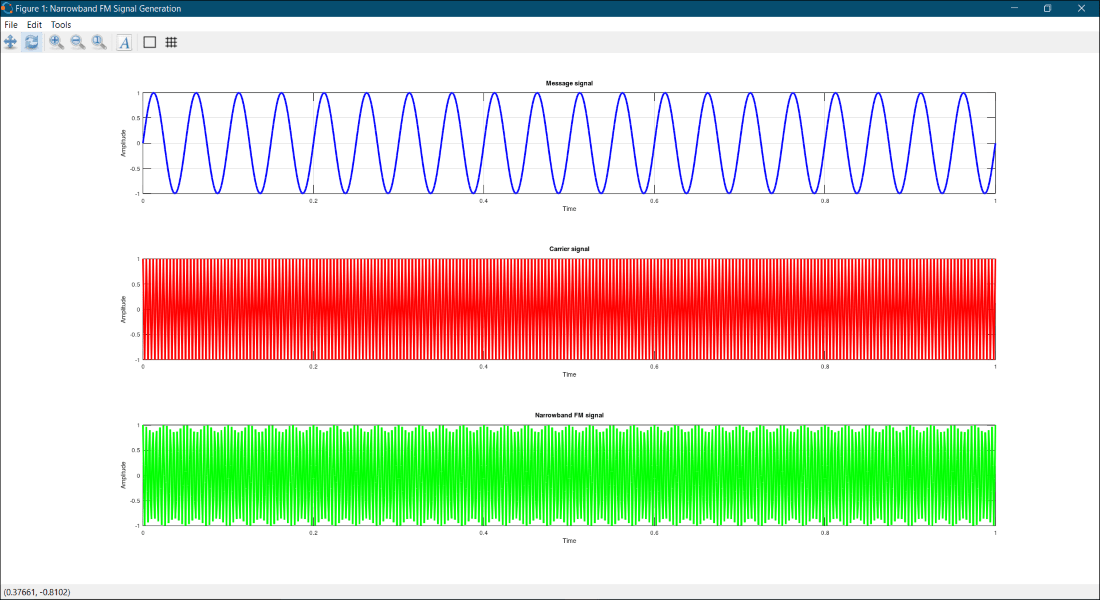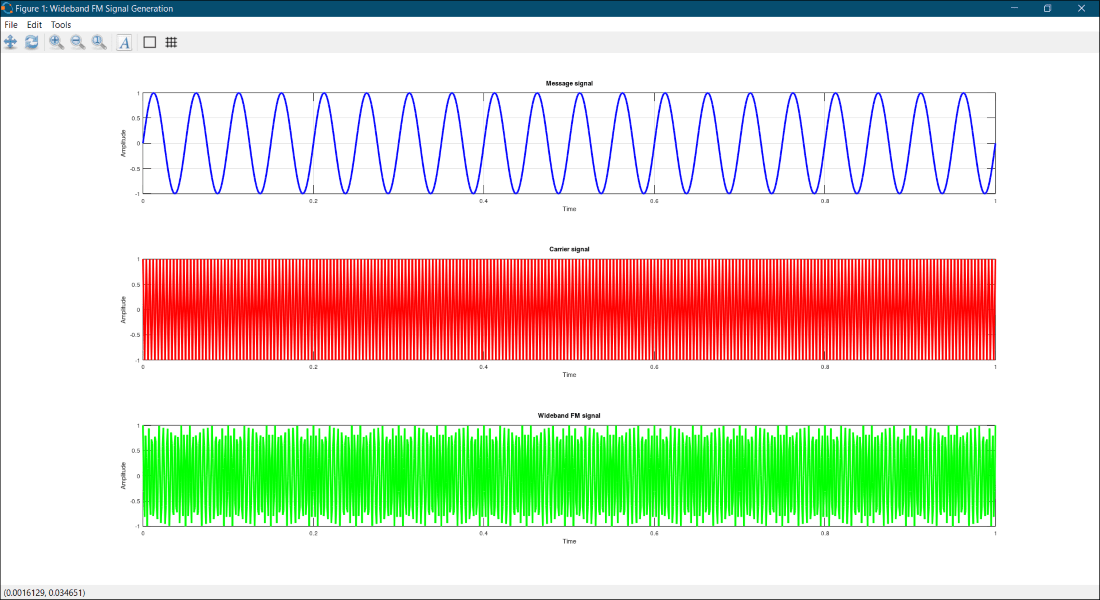# How to generate Narrowband and Wideband FM signal using GNU-Octave?

• Last Updated : 09 Jul, 2021

In this article,  we are going to discuss how to generate the Narrowband and Wideband FM signal using MATLAB.

Frequency Modulation popularly known as FM is a kind of analog modulation technique in which the frequency of the high-frequency carrier signal is varied according to the amplitude of the modulating (message) signal while the amplitude and the phase of the carrier signal remain constant or unchanged.

### Standard equation of an FM signalwhere;  fm = FM signal,

Ac = Amplitude of carrier signal,

fc = Carrier Frequency,

fm = Modulating Frequency,

t = time,  & b = Frequency modulation index.

FM signals are classified into two broad categories depending upon the value of the frequency modulation index (b):

• Narrowband FM
• Wideband FM

### Narrowband FM signal

In general, the FM signals which have their frequency modulation index (b<1) less than unity are called Narrowband FM signals. Usually, narrowband FM signals are used in low-cost two-way radio communication systems like a walkie-talkie.

To generate the narrowband FM signal, we will be using the standard equation of the FM signal and pass the value of the frequency modulation index (b) less than unity.

Example:

## Matlab

 `%  MATLAB code to generate the narrowband FM signal``clc;       ``% Clears the Command Window``clear all; ``% Clears the Workspace``close all; ``% Closes all the Windows opened by the program`` ` `% Time sampling where Step Size = 0.001``t = 0:0.001:1;``fm = 20; ``% frequency of the Message signal``fc = 250; ``% frequency of the Carrier signal`` ` `% Take the user input for the Frequency Modulation Index (b)``% (b) must be less than 1``b = input(``"Enter the value of b (<1): "``);`` ` `% Define the Message signal``% here its a Sine wave``msg = sin(2*pi*fm*t);`` ` `% Define the Carrier signal``% here it is a Cosine wave``crr = cos(2*pi*fc*t);`` ` `% Narrowband FM Signal generation``nb_fm = cos((2*pi*fc*t)+(b*sin(2*pi*fm*t)));`` ` `% Plot all the three signals``figure(``'Name'``,``'Narrowband FM Signal Generation'``);`` ` `% Message Signal``subplot(3,1,1);``plot(t, msg, ``'b'``, ``'Linewidth'``, 1.5);``title(``'Message signal'``);``xlabel(``'Time'``)``ylabel(``'Amplitude'``)``grid on;`` ` `% Carrier Signal``subplot(3,1,2);``plot(t, crr, ``'r'``, ``'Linewidth'``, 1.5);``title(``'Carrier signal'``);``xlabel(``'Time'``)``ylabel(``'Amplitude'``)``grid on;`` ` `% Narrowband FM Signal``subplot(3,1,3);``plot(t, nb_fm, ``'g'``, ``'Linewidth'``, 1.5);``title(``'Narrowband FM signal'``);``xlabel(``'Time'``)``ylabel(``'Amplitude'``)``grid on;`

Output:

`Enter the value of b (<1): 0.55`Output: Narrowband FM Signal Generation

### Wideband FM signal

Unlike the narrowband FM signals, wideband FM signals are the FM signals whose frequency modulation index (b>1) is greater than unity. Normally, wideband signals are used for high-quality broadcast transmission.

To generate the wideband FM signal, we will be using the standard equation of the FM signal and pass the value of the frequency modulation index (b) greater than unity.

Example:

## Matlab

 `%  MATLAB code to generate the wideband FM signal``clc;       ``% Clears the Command Window``clear all; ``% Clears the Workspace``close all; ``% Closes all the Windows opened by the program`` ` `% Time sampling where Step Size = 0.001``t = 0:0.001:1;`` ` `fm = 20; ``% frequency of the Message signal``fc = 250; ``% frequency of the Carrier signal`` ` `% Take the user input for the Frequency Modulation Index (b)``% (b) must be greater than 1``b = input(``"Enter the value of b (>1): "``);`` ` `% Define the Message signal``% here its a Sine wave``msg = sin(2*pi*fm*t);`` ` `% Define the Carrier signal``% here it is a Cosine wave``crr = cos(2*pi*fc*t);`` ` `% Wideband FM Signal generation``wb_fm = cos((2*pi*fc*t)+(b*sin(2*pi*fm*t)));`` ` `% Plot all the three signals``figure(``'Name'``,``'Wideband FM Signal Generation'``);`` ` `% Message Signal``subplot(3,1,1);``plot(t, msg, ``'b'``, ``'Linewidth'``, 1.5);``title(``'Message signal'``);``xlabel(``'Time'``)``ylabel(``'Amplitude'``)``grid on;`` ` `% Carrier Signal``subplot(3,1,2);``plot(t, crr, ``'r'``, ``'Linewidth'``, 1.5);``title(``'Carrier signal'``);``xlabel(``'Time'``)``ylabel(``'Amplitude'``)``grid on;`` ` `% Wideband FM Signal``subplot(3,1,3);``plot(t, wb_fm, ``'g'``, ``'Linewidth'``, 1.5);``title(``'Wideband FM signal'``);``xlabel(``'Time'``)``ylabel(``'Amplitude'``)``grid on;`

Output:

`Enter the value of b (>1): 2.5`Output: Wideband FM Signal Generation

My Personal Notes arrow_drop_up